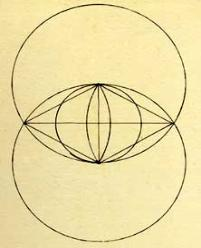.
 .
Music is composed of sounds and sounds come from
vibrations, and vibrations are deteremined by the lengths
of strings, or wavelengths in air, that translates into
cycles per second which gives us different notes.Music and         Sacred Geometry
In the process of going from One to Two, a Circle with a
diameter length has a single vibration, but when another Circle
is added interesecting it in its center, a Vesica Pisces is formed.
This is the area that it shares which looks like the female
generative organ, and from where in sacred geometry  all
things come from. It is part of the reproductive creative
process of the Lord (SEE
Golden Section Class). ....For this is
how the Lord multiplication and reproduction came about from
One to Many Vibrations that we see and feel and hear. and yet
all are in tune with the One Originator, who is the LORD, the
One God.  (SEE
Sexual Mysteries Board )
O.K. so if there was a string across the diameter of our first
circle, that could be our base frequency of 1,The second circle
makes the Vesica Pisces, and extends the distance to 1.5 times
the first. Therefore any frequency times 1.5 gives a pitch that is
in tune with the original pitch or vibration.
And then if we continue on with the construction of a new
Vesica Pisces by the mating of two circles, it makes a new
larger circle of 2.25 times larger than our original circle. And
so if we half this diamter, into a range between 1 and 2, it is
1.125. Therefore any original frequency sounds in tune with
that frequency times 1.125. And lo and behold we can go on
making twelve notes between 1 and its octave of 2. 1.125 being
the B note on the piano.
Therefore using this same process we get a table, as seen on
the left, with each of its corresponding notes that are all
harmoniously in tune with each other, and now you know why.
Circle Diameter    Ratio        Note     Hertz
1 = 1                  1                  A       440
2 = 1.5               1.5               E        659
3 = 2.25             1.125           B       494
4 = 3.375           1.69             F#      743
5 = 5.062           1.265           C#     554
6 = 7.539           1.88             G#     827
7 = 11.39           1.42             D#     622
8 = 17.08           1.06             A#     466
9 = 25.62           1.59             F        698
10=38.44           1.19             C        523
11=57.66           1.79             G        787
12=86.49           1.35             D        582
But what makes them all sound in tune when played
together ... Well, they have to be an octave of or a
ratio of the original string or wavelength. If a sound
of 110 hertz is played, it goes together harmonically
with 220 hertz and 440 hertz because they are
octaves apart. 110 x 2 = 220 etc. etc. or of 55 H
because that is half of 110 H., etc. etc.So using these seven basic step measures , each note on the piano,
has a ratio between them as follows
 .
Therefore musical notes sound in tune because they fit together in their sound waves and vibrate in unison along their
compression patterns and its all mathematical and relates back to the Original. And this gets very interesting when
you consider that our Earth has a frequency (SEE
Earth Frequency) and the Lord has His Frequencies of
transmission if we are in tune. (SEE
Crystalline Skull). We can literally hear His Music and His Voice.

IHS

David Jay Jordan